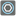• # Strange index errors

## Question related to missionEthernet Ring Dimensioning

Hi there,

Now I am facing strange Index error with following code. There is a test case without flows like "checkio(["9876543210"])" and I could not pass this case due to strange index error. Actually, this case does not provide parameter named "flows" therefore I put a IF statement (if len(flows) > 0:), however, index error occurred under the IF block. More precisely, if e.endswith(f): 6 lines below the if statement. I don't understand why this index error occurred even though the statement should not go through for this test case without parameter flows. Could someone give me advice to move forward?

```ETHERNET = (100, 40, 10, 1, 0.1) # Ethernet bandwidth capacity in Gbps

def checkio(ring, *flows):

if len(flows) == 0:
flows = ring[1:]
ring = ring

if not isinstance(ring, str):
ring = str(ring)

path_list = []

# Get path list
for i in range(len(ring)-1):
path_list.append(ring[i]+ring[i+1])
path_list.append(ring[-1]+ring)
flow_dict = {}
#print(path_list)

if len(flows) > 0:
for f in flows:
# Forward
for e in path_list:
if e.startswith(f):
s_sec = e
if e.endswith(f):
e_sec = e

# These worked fine in my local environment but not in checkio. (IndexError: list index out of range)
#s_loc = path_list.index([a for a in path_list if a.startswith(f)])
#e_loc = path_list.index([a for a in path_list if a.endswith(f)])
# Alternative coding
s_loc = path_list.index(s_sec)
e_loc = path_list.index(e_sec)

if e_loc >= s_loc:
distance = e_loc - s_loc
else:
distance = len(path_list) - e_loc + s_loc - 1
# backward case
if distance + 1 > len(path_list) / 2:
distance = (len(path_list) - distance - 2) * -1 #negative if backward
s_loc -= 1
if s_loc == -1:
s_loc = len(path_list) - 1
e_loc += 1
if e_loc >= len(path_list):
e_loc = 0

for i in range(abs(distance) + 1):
if distance >= 0:
location = s_loc + i
if location >= len(path_list):
location -= len(path_list)
if path_list[location] in flow_dict:
flow_dict[path_list[location]] += f
else:
flow_dict[path_list[location]] = f
else:
location = s_loc - i
if location < 0:
location += len(path_list)
if path_list[location] in flow_dict:
flow_dict[path_list[location]] += f
else:
flow_dict[path_list[location]] = f

#print(flow_dict)
result_list = [0, 0, 0, 0, 0]
for elem in flow_dict.items():
for bw in range(1, 6):
if ETHERNET[-bw] >= elem:
result_list[-bw] += 1
break
# take care of extra large numbers
if elem > ETHERNET:
result_list += elem // ETHERNET
if elem % ETHERNET != 0:
result_list += 1

print(result_list)
return result_list

if __name__ == '__main__':
# These "asserts" are used only for self-checking and not necessary for auto-testing
assert checkio("AEFCBG", ("AC", 5), ("EC", 10), ("AB", 60)) == [2, 2, 1, 0, 0]
assert checkio("ABCDEFGH", ("AD", 4)) == [0, 0, 3, 0, 0]
assert checkio("ABCDEFGH", ("AD", 4), ("EA", 41)) == [4, 0, 3, 0, 0]
assert checkio(["9876543210"]) == [0, 0, 0, 0, 0]
```9